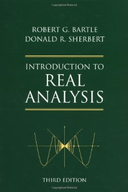Introduction To Real Analysis - 3 Edition - Chapter 3.4 - Problem 3
Register Now

Join StudySoup

Get Full Access to Introduction To Real Analysis - 3 Edition - Chapter 3.4 - Problem 39780471321484

# Let Un) be the Fibonacci sequence of Example 3.I.2(d), and let xn := fn+,/fn. Given that

Introduction to Real Analysis | 3rd Edition

Problem 3

Let Un) be the Fibonacci sequence of Example 3.I.2(d), and let xn := fn+,/fn. Given that lim(x n)._=. L exists, determine the value of L.

Accepted Solution
Step-by-Step Solution:
Step 1 of 3

PAM 2000 McDermott Spring 2016 Prelim 2 Study Guide Lecture and Discussion Notes LECTURE NOTES:  Economics of PATENT LAW o pros: incentivizes innovation o cons: creates monopolies  inefficient market o Stackelberg model and first mover advantage o alternative  shorter patent life  government projects (drug research)  government grants/subsidies o pharmaceutical industry  expensive to generate new drugs  patent: get a monopoly for 20 years o cases  A: with patent, unrestricted  B: no patent  C: with patent but with price regulation 

###### Chapter 3.4, Problem 3 is Solved

Step 2 of 3

Step 3 of 3

Unlock Textbook Solution